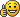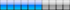## SINE FUNCTION ADDS MOTION TO SCENES

### SINE FUNCTION ADDS MOTION TO SCENES

I make use of a very simple but effective script in many of my scenes for causing geometries to rythmically and smoothly rock back and forth, and I wanted to pass this on to others who may not be aware of this. It's especially handy for making clouds, fog, and water move very slowly and naturally back and forth. You can also change a couple of numbers and make your objects oscillate very fast or even vibrate like crazy! Here is how it's done.....

Many of us who learned basic geometry or trigonometry in high school and college are familiar with the SINE and COSINE functions. When you plot these functions on a graphing calculator or a computer, you know that the result is a wavy line that has a range of values on the Y-axis of between -1 and +1. The plotted waveform can look boring and useless on the screen of a calculator, but that same waveform can be used to do intersting things in Algodoo! The Thyme function that returns the sine of an angle is math.sin(n). And a similar function that returns the cosine of an angle is math.cos(n). If you put either of these functions in a loop, or in a script that gets evaluated repeatedly (such as OnCollide or OnHitByLaser, AND if (n) is a continuously incrementing value (such as sim.time or system.time) then the script will produce output values with magnitudes that rise and fall with a sinusoidal shape. Those values can be used to drive the motion of anything in Algodoo that can be influenced by changing values. You can make a geometry swing back and forth in the X direction, up and down in the Y direction, or you can make a motor turn clockwise and counterclockwise.

The math.sin and math.cos functions are similar, but with equal input vlues the magnitudes of the output values are 90 degrees out of phase with each other. That fact can be used for producing believable and realistic-looking effects.

Please refer to my scene "SINE FUNCTION MOTION - Tutorial" for the following explanations:

I want to start by looking at Panel - 4, which shows a traditional plot of the SINE and COSINE functions. You can see that the waveforms are out of phase with each other (90 degrees to be exact). I make use of that in an interesting way that I discovered while experimenting with textures. I found that I can get a nice effect by slowly waving a geometry in the X direction containing a texture of a tree or a plant or grass, etc, with the math.sin function, AND by waving the Xoffset of the texture image with the math.cos function. Refer to Panel - 5 for links to a couple of scenes in which I use this technique.

If you compare the movements of the two red cars (in Panels 2 and 3), you will note that the car in Panel - 2 moves more slowly than the car in Panel -3, and the distance that it moves is farther. Those differences are controlled by two values in the script that generates the motion.

Refer to Panel - 1 for the following explanation:
This is the right side of the equation that uses the SINE or COSINE function to make the desired motions of the objects in your scene. The left side of the equation is simply a variable that you determine will create the motion (look at my sample scenes for a few examples). For those red cars, I created a local variable, _Fsin, which I use as an offset for pos(0). As the equation gets evaluated with each simulation frame, the resulting sinusoidal waveform offsets the current X axis of the car, giving it that rythmic swaying back and forth. As shown in the panel, the constant that increases or decreases the magnitude of the sim.time value (in this example it is 3) affects the FREQUENCY of oscillation. The constant that increases or decreases the output SINE or COSINE value (in this example it is 2) affects the AMPLITUDE of oscillation. You can either MULTIPLY or DIVIDE those constants, depending on the type of motion that you are looking for. My best advice for you is to EXPERIMENT with these values and see what they do, and how they affect the motion of the object.

There are other math functions, such as math.tan, which produce much different waveforms than sine and cosine, but that goes beyond the scope of this tutorial. If you want to experiment with other functions, or even create some functions of your own, go ahead and have fun because you can't harm anything. You just might create a motion that no one has ever seen before in Algobox!Rating:Filesize: 331.68 kB
Ratings: 2Xray

Posts: 489
Joined: Sun Jun 17, 2012 6:12 am
Location: USA

### Re: SINE FUNCTION ADDS MOTION TO SCENES

PostStep sctipt:
Code: Select all
`(e)=>{e.this.vel := [0, (math.sin(sim.time * 10))]}`
Enough Said.
My signature is worth a lot of money, So I'm gonna put this stamp instead:
[\$20 PER AUTOGRAPH]

Posts: 26
Joined: Tue Aug 17, 2010 7:37 am

### Re: SINE FUNCTION ADDS MOTION TO SCENES

Xray
Nice!
thats precisely what i was searching for!!DrAgon

Posts: 29
Joined: Tue Feb 12, 2013 4:03 pm